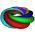Wednesday, November 14, 2012

Algebra II (5) Assessment Data #3 (Quadratics)

November 14, 2012

I shared this data on our quadratics quiz with students in class. Here are the results.

The biggest mistake was on # 12 and #16.  Remember in factored form you must set each term equal to zero and solve.

The roots of y=(x-3)(x+2) are 3 and -2.

The roots of y=(2x-1)(x+2) are 1/2 and -2.

In both cases each factor is set equal to zero as in (2x-1)=0 and x+2=0.

Average was an 89% and median was a 91%.

My Best,

Mr. Morrissey

1 comment:

1.This comment has been removed by a blog administrator.